Back to index

Abstract

Digital video can suffer from a plethora of visual artifacts caused by lossy compression, transmission, quantization, and even errors in the mastering process. These artifacts include, but are not limited to, banding, aliasing, loss of detail and sharpness (blur), discoloration, halos and other edge artifacts, and excessive noise.
Since many of these defects are rather common, filters have been created to remove, minimize, or at least reduce their visual impact on the image. However, the stronger the defects in the source video are, the more aggressive filtering is needed to remedy them, which may induce new artifacts.

In order to avoid this, masks are used to specifically target the affected scenes and areas while leaving the rest unprocessed. These masks can be used to isolate certain colors or, more importantly, certain structural components of an image. Many of the aforementioned defects are either limited to the edges of an image (halos, aliasing) or will never occur in edge regions (banding). In these cases, the unwanted by-products of the respective filters can be limited by only applying the filter to the relevant areas. Since edge masking is a fundamental component of understanding and analyzing the structure of an image, many different implementations were created over the past few decades, many of which are now available to us.

In this article, I will briefly explain and compare different ways to generate masks that deal with the aforementioned problems.

Theory, examples, and explanations

Most popular algorithms try to detect abrupt changes in brightness by using convolutions to analyze the direct neighbourhood of the reference pixel. Since the computational complexity of a convolution is 0(n2) (where n is the radius), the the radius should be as small as possible while still maintaining a reasonable level of accuracy. Decreasing the radius of a convolution will make it more susceptible to noise and similar artifacts.

Most algorithms use 3x3 convolutions, which offer the best balance between speed and accuracy. Examples are the operators proposed by Prewitt, Sobel, Scharr, and Kirsch. Given a sufficiently clean (noise-free) source, 2x2 convolutions can also be used[src], but with modern hardware being able to calculate 3x3 convolutions for HD video in real time, the gain in speed is often outweighed by the decreased accuracy.

To better illustrate this, I will use the Sobel operator to compute an example image.
Sobel uses two convolutions to detect edges along the x and y axes. Note that you either need two separate convolutions per axis or one convolution that returns the absolute values of each pixel, rather than 0 for negative values.

 -1 -2 -1 0 0 0 1 2 1 -1 0 1 -2 0 2 -1 0 1

Every pixel is set to the highest output of any of these convolutions. A simple implementation using the Convolution function of Vapoursynth would look like this:

``````def sobel(src):
sx = src.std.Convolution([-1, -2, -1, 0, 0, 0, 1, 2, 1], saturate=False)
sy = src.std.Convolution([-1, 0, 1, -2, 0, 2, -1, 0, 1], saturate=False)
return core.std.Expr([sx, sy], 'x y max')``````
Fortunately, Vapoursynth has a build-in Sobel function `core.std.Sobel`, so we don't even have to write our own code.

Hover over the following image to see the Sobel edge mask.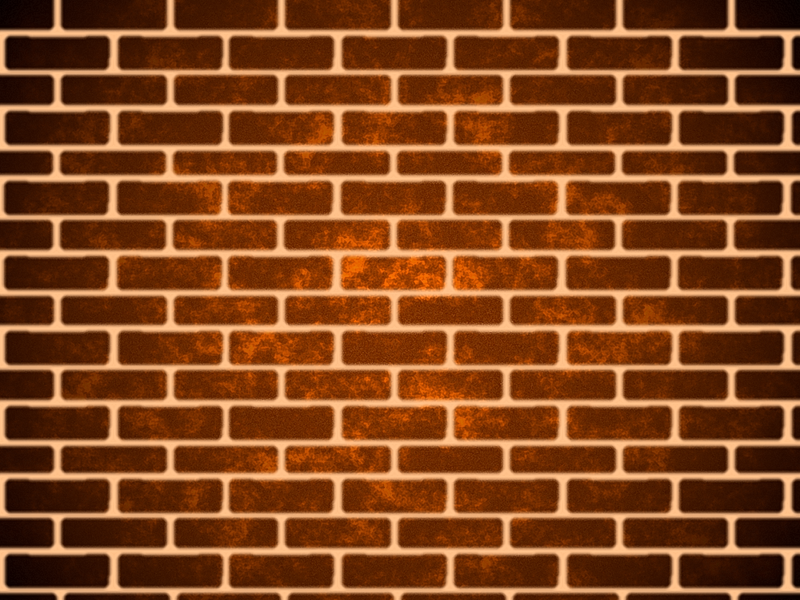Of course, this example is highly idealized. All lines run parallel to either the x or the y axis, there are no small details, and the overall complexity of the image is very low.

Using a more complex image with blurrier lines and more diagonals results in a much more inaccurate edge mask.A simple way to greatly improve the accuracy of the detection is the use of 8-connectivity rather than 4-connectivity. This means utilizing all eight directions of the Moore neighbourhood, i.e. also using the diagonals of the 3x3 neighbourhood.
To achieve this, I will use a convolution kernel proposed by Russel A. Kirsch in 1970[src].

5 5 5 -3 0 -3 -3 -3 -3
This kernel is then rotated in increments of 45° until it reaches its original position.
Since Vapoursynth does not have an internal function for the Kirsch operator, I had to build my own; again, using the internal convolution.
``````def kirsch(src):
kirsch1 = src.std.Convolution(matrix=[ 5,  5,  5, -3,  0, -3, -3, -3, -3])
kirsch2 = src.std.Convolution(matrix=[-3,  5,  5,  5,  0, -3, -3, -3, -3])
kirsch3 = src.std.Convolution(matrix=[-3, -3,  5,  5,  0,  5, -3, -3, -3])
kirsch4 = src.std.Convolution(matrix=[-3, -3, -3,  5,  0,  5,  5, -3, -3])
kirsch5 = src.std.Convolution(matrix=[-3, -3, -3, -3,  0,  5,  5,  5, -3])
kirsch6 = src.std.Convolution(matrix=[-3, -3, -3, -3,  0, -3,  5,  5,  5])
kirsch7 = src.std.Convolution(matrix=[ 5, -3, -3, -3,  0, -3, -3,  5,  5])
kirsch8 = src.std.Convolution(matrix=[ 5,  5, -3, -3,  0, -3, -3,  5, -3])
return core.std.Expr([kirsch1, kirsch2, kirsch3, kirsch4, kirsch5, kirsch6, kirsch7, kirsch8],
'x y max z max a max b max c max d max e max')``````

It should be obvious that the cheap copy-paste approach is not acceptable to solve this problem. Sure, it works, but I'm not a mathematician, and mathematicians are the only people who write code like that. Also, yes, you can pass more than three clips to sdt.Expr, even though the documentation says otherwise.
Or maybe my limited understanding of math (not being a mathematician, after all) was simply insufficient to properly decode “Expr evaluates an expression per pixel for up to 3 input clips.”

Anyway, let's try that again, shall we?
``````def kirsch(src: vs.VideoNode) -> vs.VideoNode:
w = *3 + [-3]*5
weights = [w[-i:] + w[:-i] for i in range(4)]
c = [core.std.Convolution(src, (w[:4]++w[4:]), saturate=False) for w in weights]
return core.std.Expr(c, 'x y max z max a max')``````

Much better already. Who needed readable code, anyway?

If we compare the Sobel edge mask with the Kirsch operator's mask, we can clearly see the improved accuracy. (Hover=Kirsch)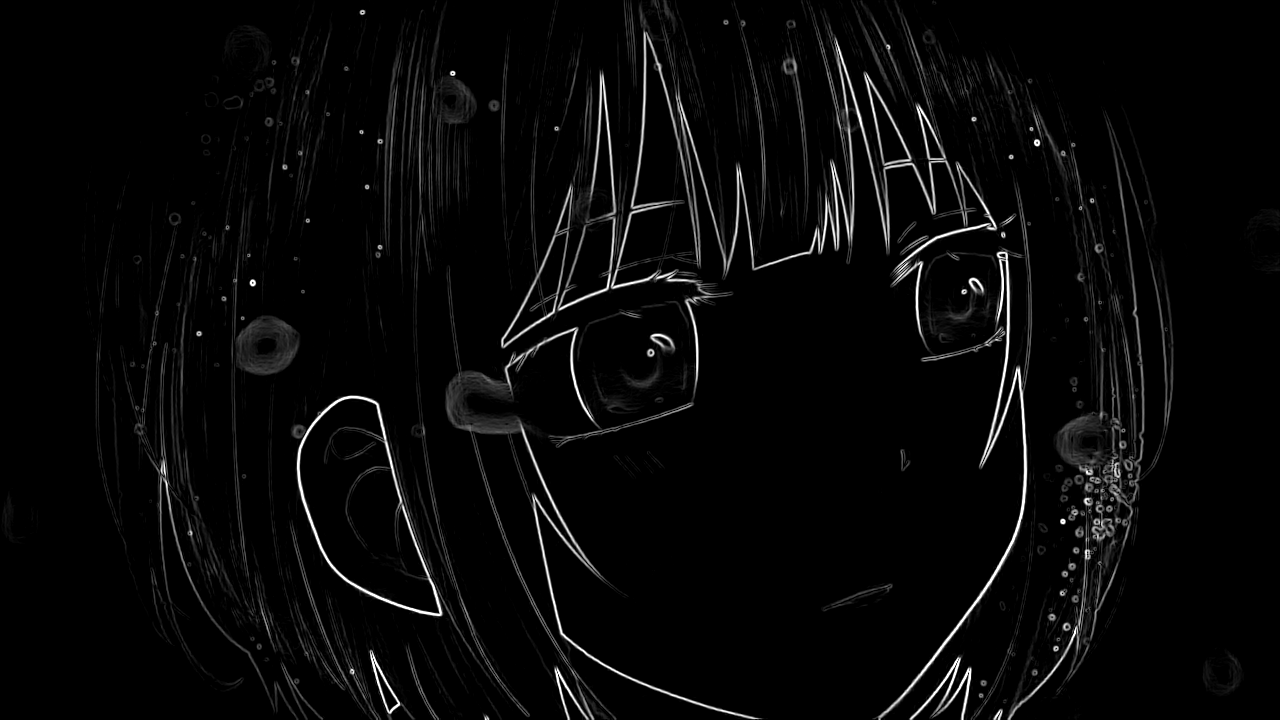The higher overall sensitivity of the detection also results in more noise being visible in the edge mask. This can be remedied by denoising the image prior to the analysis.
The increase in accuracy comes at an almost negligible cost in terms of computational complexity. About 175 fps for 8-bit 1080p content (luma only) compared to 215 fps with the previously shown sobel ‘implementation’. The internal Sobel filter is not used for this comparison as it also includes a high- and lowpass function as well as scaling options, making it slower than the Sobel function above. Note that many of the edges are also detected by the Sobel operator, however, these are very faint and only visible after an operation like std.Binarize.

Note: It has been pointed out to me that my implementation of the Kirsch edgemask is actually incorrect. I have since corrected this, so if you’re using the latest version from Github, you should already have it. The comparison images above use the incorrect implementation, but the difference is still very visible.

A more sophisticated way to generate an edge mask is the TCanny algorithm which uses a similar procedure to find edges but then reduces these edges to 1 pixel thin lines. Optimally, these lines represent the middle of each edge, and no edge is marked twice. It also applies a gaussian blur to the image to eliminate noise and other distortions that might incorrectly be recognized as edges. The following example was created with TCanny using these settings: ```core.tcanny.TCanny(op=1, mode=0)```. op=1 uses a modified operator that has been shown to achieve better signal-to-noise ratios[src].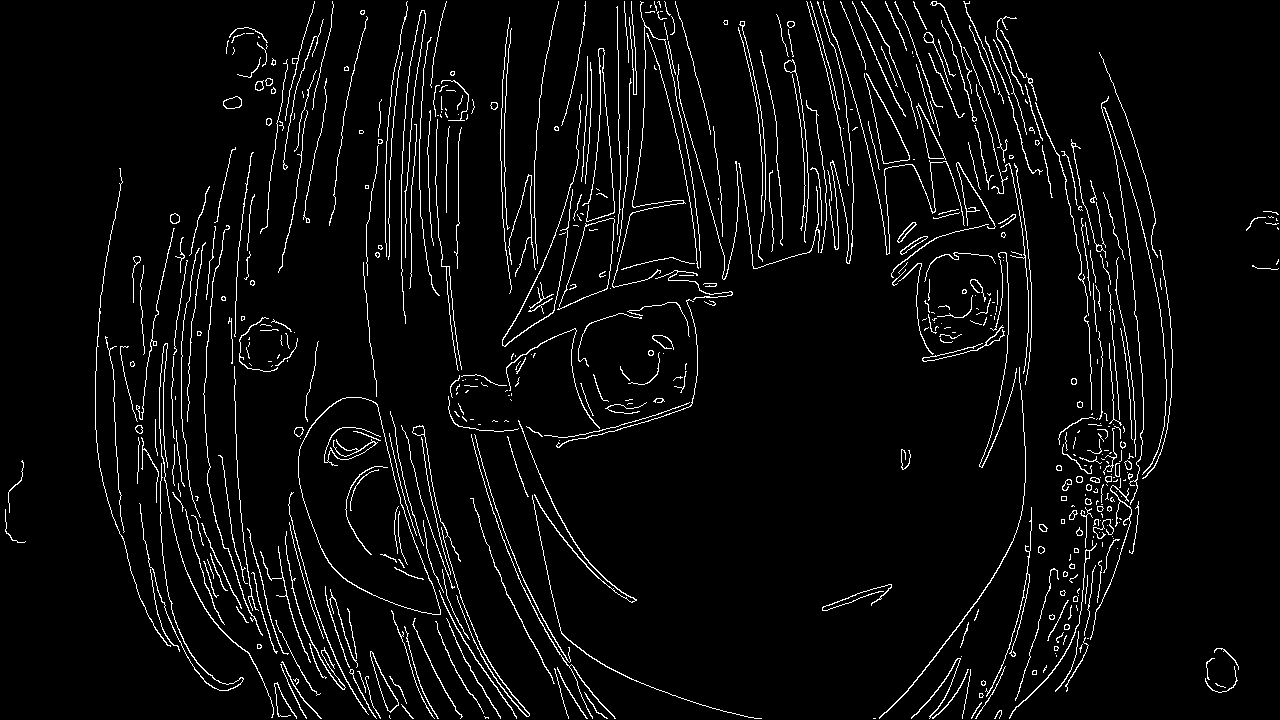Since I've already touched upon bigger convolutions earlier without showing anything specific, here is an example of the things that are possible with 5x5 convolutions.
``````src.std.Convolution(matrix=[1,  2,  4,  2, 1,
2, -3, -6, -3, 2,
4, -6,  0, -6, 4,
2, -3, -6, -3, 2,
1,  2,  4,  2, 1], saturate=False)``````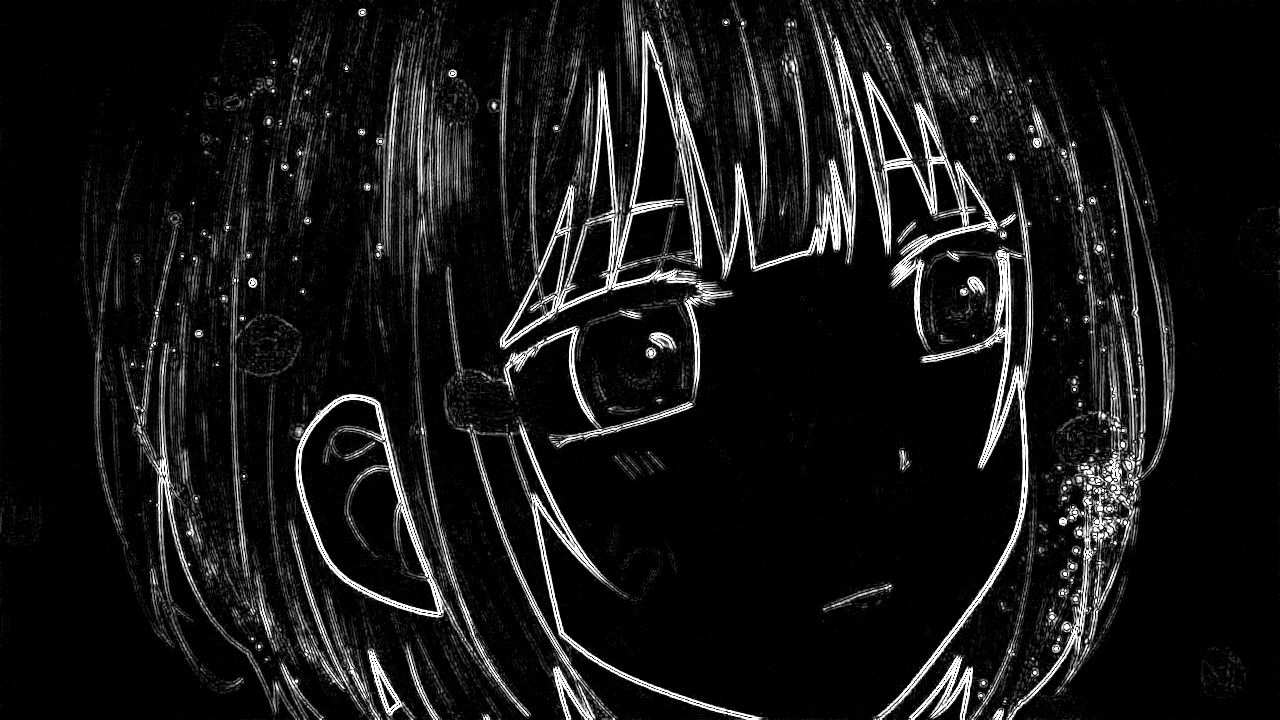This was an attempt to create an edge mask that draws around the edges. With a few modifications, this might become useful for halo removal or edge cleaning. (Although something similar (probably better) can be created with a regular edge mask, std.Maximum, and std.Expr)

Now that we've established the basics, let's look at real world applications. Since 8-bit video sources are still everywhere, barely any encode can be done without debanding. As I've mentioned before, restoration filters can often induce new artifacts, and in the case of debanding, these artifacts are loss of detail and, for stronger debanding, blur. An edge mask could be used to remedy these effects, essentially allowing the debanding filter to deband whatever it deems necessary and then restoring the edges and details via std.MaskedMerge.

GradFun3 internally generates a mask to do exactly this. f3kdb, the other popular debanding filter, does not have any integrated masking functionality.

Consider this image:As you can see, there is quite a lot of banding in this image. Using a regular debanding filter to remove it would likely also destroy a lot of small details, especially in the darker parts of the image.
Using the Sobel operator to generate an edge mask yields this (admittedly rather disappointing) result: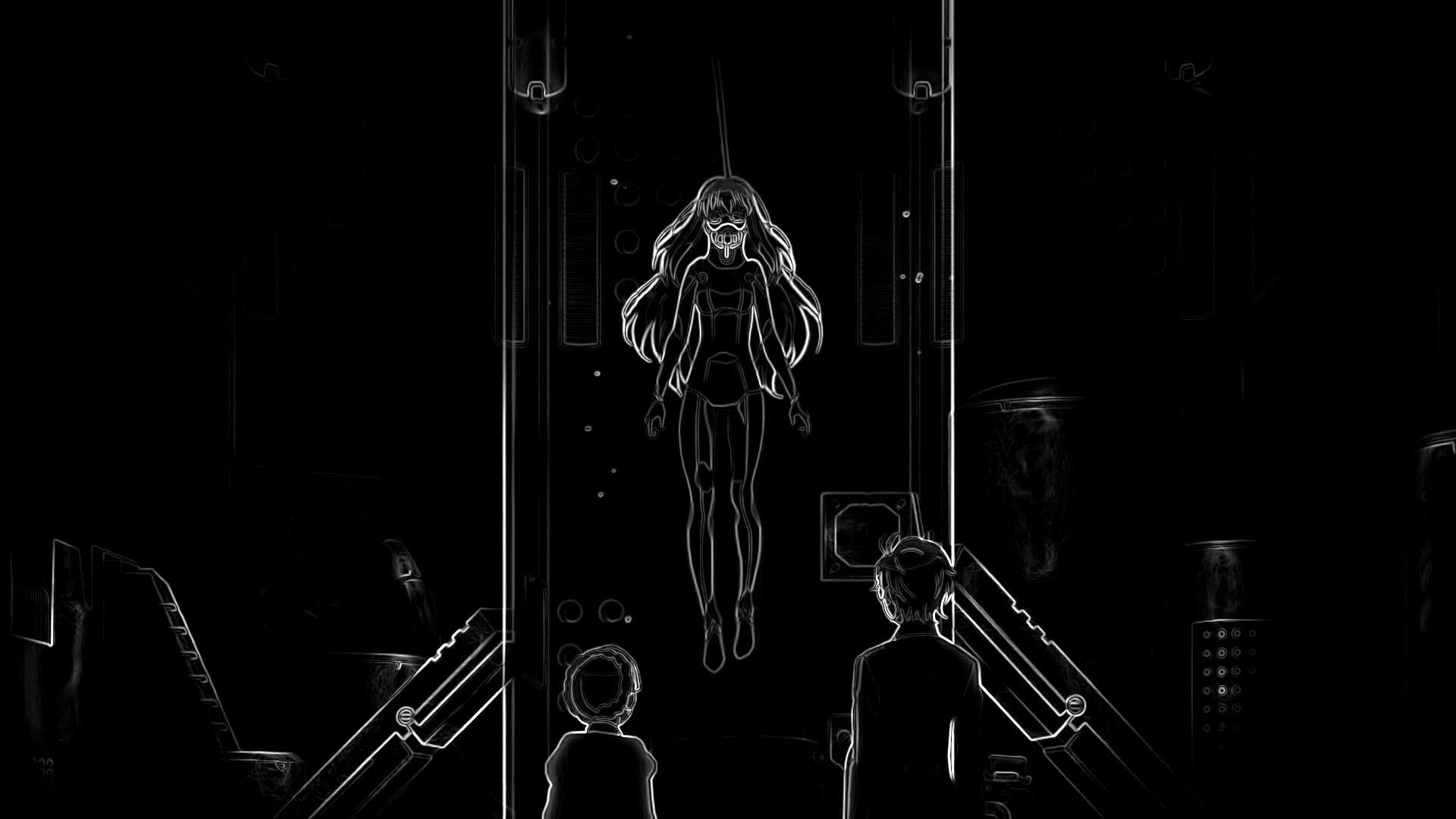In order to better recognize edges in dark areas, the retinex algorithm can be used for local contrast enhancement.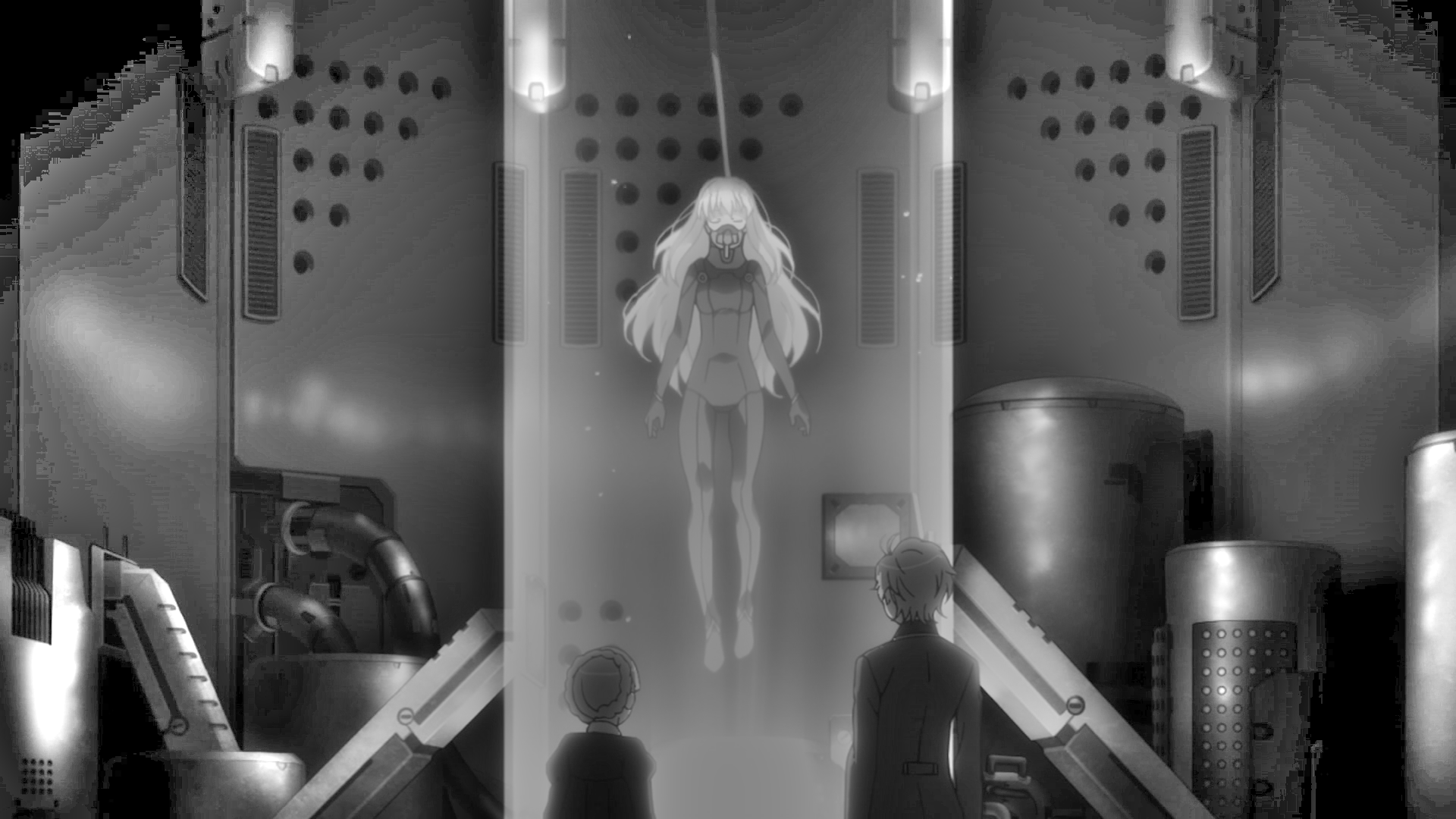The image after applying the retinex filter, luma only.

We can now see a lot of information that was previously barely visible due to the low contrast. One might think that preserving this information is a vain effort, but with HDR-monitors slowly making their way into the mainstream and more possible improvements down the line, this extra information might be visible on consumer grade screens at some point. And since it doesn't waste a noticeable amount of bitrate, I see no harm in keeping it.

Using this newly gained knowledge, some testing, and a little bit of magic, we can create a surprisingly accurate edge mask.
``````def retinex_edgemask(luma, sigma=1):
ret = core.retinex.MSRCP(luma, sigma=[50, 200, 350], upper_thr=0.005)
return core.std.Expr([kirsch(luma), ret.tcanny.TCanny(mode=1, sigma=sigma).std.Minimum(
coordinates=[1, 0, 1, 0, 0, 1, 0, 1])], 'x y +')``````

Using this code, our generated edge mask looks as follows: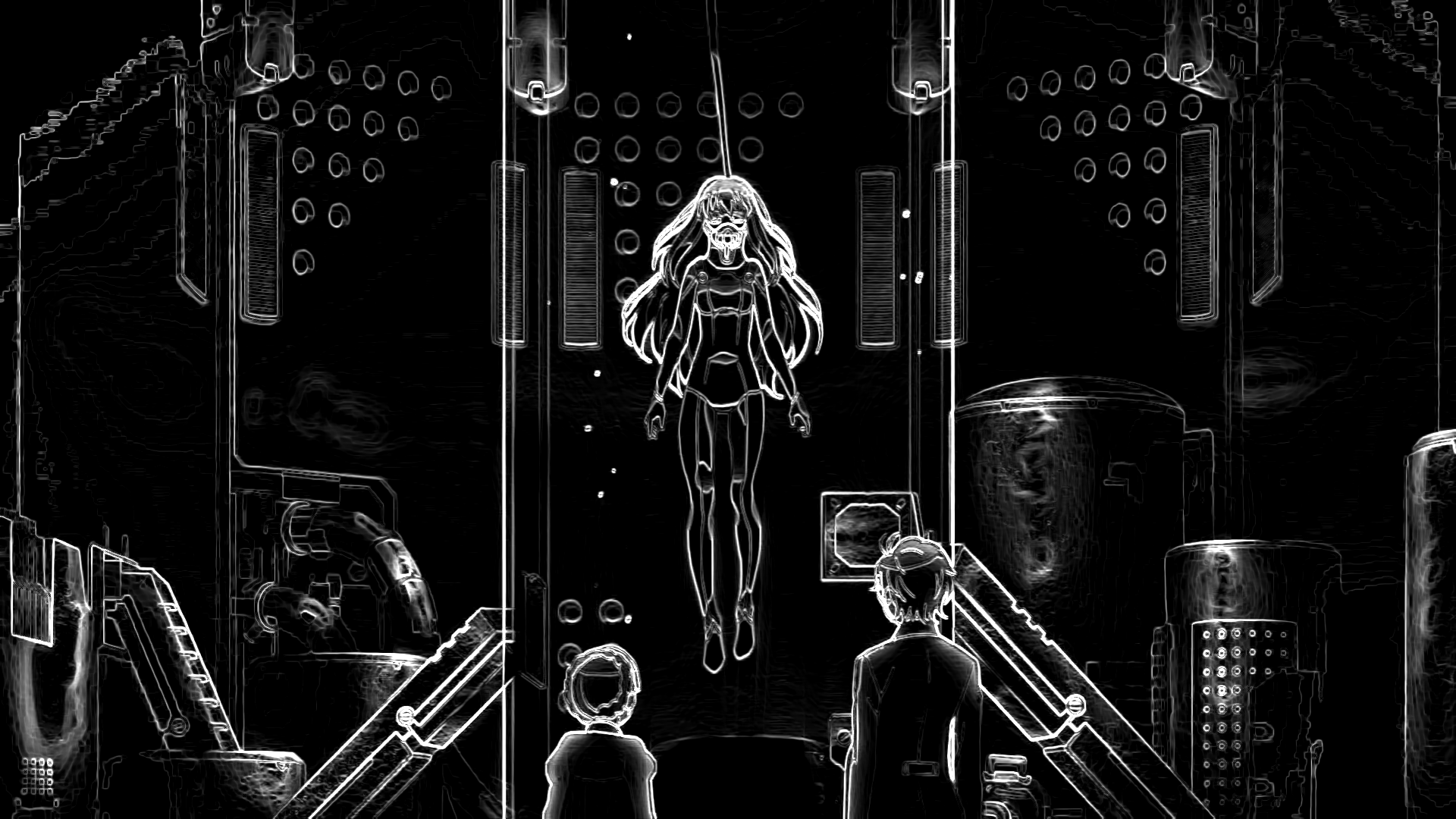By using std.Binarize (or a similar lowpass/highpass function) and a few std.Maximum and/or std.Inflate calls, we can transform this edgemask into a more usable detail mask for our debanding function or any other function that requires a precise edge mask.

Performance

Most edge mask algorithms are simple convolutions, allowing them to run at over 100 fps even for HD content. A complex algorithm like retinex can obviously not compete with that, as is evident by looking at the benchmarks. While a simple edge mask with a Sobel kernel ran consistently above 200 fps, the function described above only procudes 25 frames per second. Most of that speed is lost to retinex, which, if executed alone, yields about 36.6 fps. A similar, albeit more inaccurate, way to improve the detection of dark, low-contrast edges would be applying a simple curve to the brightness of the image.
``bright = core.std.Expr(src, 'x 65535 / sqrt 65535 *')``
This should (in theory) improve the detection of dark edges in dark images or regions by adjusting their brightness as shown in this curve:## Conclusion

Edge masks have been a powerful tool for image analysis for decades now. They can be used to reduce an image to its most essential components and thus significantly facilitate many image analysis processes. They can also be used to great effect in video processing to minimize unwanted by-products and artifacts of more agressive filtering. Using convolutions, one can create fast and accurate edge masks, which can be customized and adapted to serve any specific purpose by changing the parameters of the kernel. The use of local contrast enhancement to improve the detection accuracy of the algorithm was shown to be possible, albeit significantly slower.

``````# Quick overview of all scripts described in this article:
################################################################

# Use retinex to greatly improve the accuracy of the edge detection in dark scenes.
# draft=True is a lot faster, albeit less accurate
def retinex_edgemask(src: vs.VideoNode, sigma=1, draft=False) -> vs.VideoNode:
core = vs.get_core()
src = mvf.Depth(src, 16)
luma = mvf.GetPlane(src, 0)
if draft:
ret = core.std.Expr(luma, 'x 65535 / sqrt 65535 *')
else:
ret = core.retinex.MSRCP(luma, sigma=[50, 200, 350], upper_thr=0.005)
mask = core.std.Expr([kirsch(luma), ret.tcanny.TCanny(mode=1, sigma=sigma).std.Minimum(
coordinates=[1, 0, 1, 0, 0, 1, 0, 1])], 'x y +')

# Kirsch edge detection. This uses 8 directions, so it's slower but better than Sobel (4 directions).
def kirsch(src: vs.VideoNode) -> vs.VideoNode:
core = vs.get_core()
w = *3 + [-3]*5
weights = [w[-i:] + w[:-i] for i in range(4)]
c = [core.std.Convolution(src, (w[:4]++w[4:]), saturate=False) for w in weights]
return core.std.Expr(c, 'x y max z max a max')

# should behave similar to std.Sobel() but faster since it has no additional high-/lowpass or gain.
# the internal filter is also a little brighter
def fast_sobel(src: vs.VideoNode) -> vs.VideoNode:
core = vs.get_core()
sx = src.std.Convolution([-1, -2, -1, 0, 0, 0, 1, 2, 1], saturate=False)
sy = src.std.Convolution([-1, 0, 1, -2, 0, 2, -1, 0, 1], saturate=False)
return core.std.Expr([sx, sy], 'x y max')

# a weird kind of edgemask that draws around the edges. probably needs more tweaking/testing
# maybe useful for edge cleaning?
def bloated_edgemask(src: vs.VideoNode) -> vs.VideoNode:
return src.std.Convolution(matrix=[1,  2,  4,  2, 1,
2, -3, -6, -3, 2,
4, -6,  0, -6, 4,
2, -3, -6, -3, 2,
1,  2,  4,  2, 1], saturate=False)``````
Some of the functions described here have been added to my script collection on Github